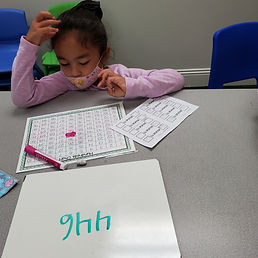## Ms. Raejean

### Target 1​

###### Lesson Type:

Continuation

Number Operation

:

Integer Composition

Use place-value understandings to round whole numbers to the nearest ten or 100.

###### 1:

Use understanding of how to round two-digit numbers and expand the strategy to three-digit numbers.

###### 2:

Understand that rounding identifies the multiple of 10 or 100 that is closest to the given number.

###### 3:

Understand that the previous place (one place smaller) is the key digit to evaluate to determine whether a number is rounded up or down.

3rd

###### Vocabulary:

Rounding, Digit, Multiples, "Nearest Ten," "Nearest Hundred"

Activities:

We reviewed rounding 2- and 3-digit numbers to the nearest ten. Then students applied their understanding to round 3-digit numbers to the nearest hundred. It was helpful to list the multiples of 100 to find the 2 nearest hundreds. We focused on "which hundred is the number closest to"?### Home Exploration

###### Guiding Questions:## Absent Students:

### Target 2

:

###### 1:

Estimate quantities and use one-to-one correspondence to check the accuracy of the estimate.

###### 2:

Develop a strategy for estimating quantities (i.e., developing a unit of measurement for estimating – if this is what 10 units looks like this must be close to 40 units).

###### 3:

Understand that rounding and estimation are a way to quickly check the accuracy of computations.

###### 4:

Understand that you can use estimation when an exact answer is not needed.

3rd

###### Vocabulary:

Estimation, Estimate, Base-Ten Blocks (Rods & Units), "Friendly Number" (Ex. Multiple of 10)

Activities:

• The term "estimation" was introduced to students. When we estimate, we change the given numbers to "friendly," easy numbers to add. During today's practice, the friendly numbers were multiples of ten. Students used base-ten blocks to help estimate the sum of 2 addends. Then students used the estimate to check the reasonableness of the actual answer. Estimations are also useful when we don't need the exact answer.

Ex. 14 + 27. When looking at the base-ten blocks, students could see that 14 is made with 1 ten and 4 ones. It is not close to making another ten, so 14 is about 10. Similarly, students could see 27 is made with 2 tens and 7 ones. It is close to making another ten, so 27 is about 30. 10 + 30 = 40 compared to 14 + 27 = 41. The answer is reasonable.### Home Exploration

###### Guiding Questions:### Target 3

:

###### 1:

Understand that a graph is a visual representation of data.

3rd

###### Vocabulary:

Tally Marks, Bar Graph

Activities:

We used the data from last week - tally marks that represent the amount of each item - to make a bar graph. Bar graphs help to organize the information so it's easy to read.### Home Exploration